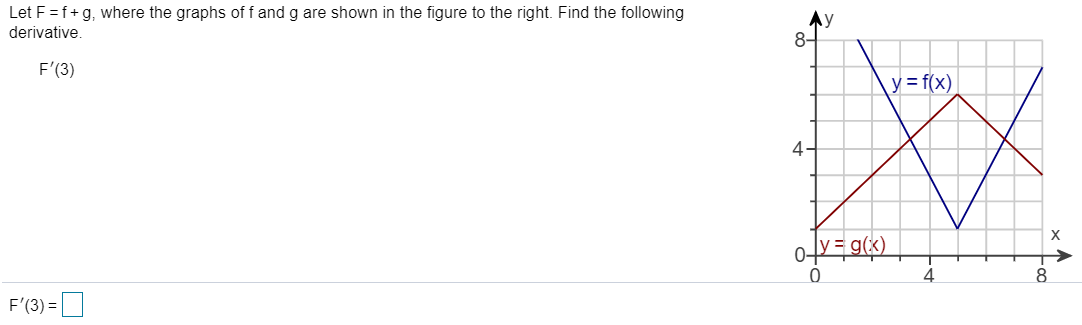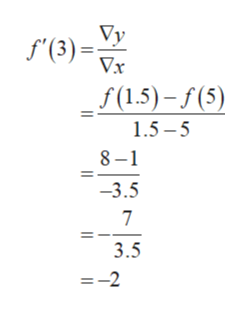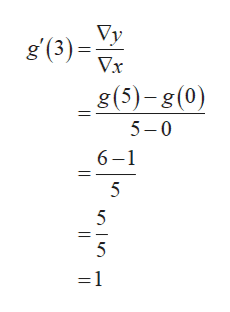Let F f+g, where the graphs of f and g are shown in the figure to the right. Find the followingderivative.F'(3)y=f(x)4y = gxF'(3)

Questionhelp_outlineImage TranscriptioncloseLet F f+g, where the graphs of f and g are shown in the figure to the right. Find the following derivative. F'(3) y=f(x) 4 y = gx F'(3) fullscreen
Step 1

It is known that, the slope of the function at a point is equal to the derivative of the function at the same point.

The derivative of the function f(x) at x = 3 is calculated as follows.help_outlineImage TranscriptioncloseVy f(3)x f(1.5)-f(5) 1.5-5 8-1 -3.5 7 3.5 =-2 l fullscreen
Step 2

The derivative of the function g(x) at x = 3 i...help_outlineImage Transcriptioncloseg (3) Vy Vx g (5)-g(0) 5 0 6 1 5 5 =1 fullscreen

Want to see the full answer?

See Solution

Want to see this answer and more?

Our solutions are written by experts, many with advanced degrees, and available 24/7

See Solution
Tagged in

Derivative